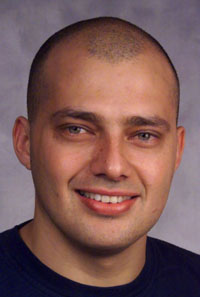• Jan 1, 1900
(b.) - ?

#### Bio/Description

Reingold was cited for finding a solution to a more than 25-year quest by expert theoretical computer science researchers and others. He addressed the problem of finding paths to connect vertices in undirected graphs (i.e. finding paths in a three dimensional maze). The time complexity of this key graph problem has been well understood for decades. Reingold's theorem resolves the memory-complexity of the problem by showing that connectivity in undirected graphs admits an extremely memory-efficient algorithm. The memory used by Reingold's algorithm is comparable to the memory needed to store simply the name of a single vertex of the graph. One of the most important consequences of this theorem is demonstrating the equivalence of two complexity classes (i.e. sets of computational problems with the same bounds on time and space) known as SL and L (Symmetric Logspace and Deterministic Logspace). This fundamental result greatly advances the understanding of the power of nondeterminism and randomization over deterministic memory-bounded computation.
• Date of Birth:

Jan 1, 1900
• Gender:

Male
• Noted For:

Author of Reingold's Algorithm relating to efficiency of memory complexity
• Category of Achievement: